• 検索結果がありません。

# density matrix renormalization group reference function

N/A
N/A
Protected

シェア "density matrix renormalization group reference function"

Copied!
244
0
0

The connectivity of the CASPT2 framework carries the internally contracted (IC) representation of the anzatz wave function originally proposed by Meyer . The IC basis is an alternative constitutional unit of the many-body wave function for the Slater determinant.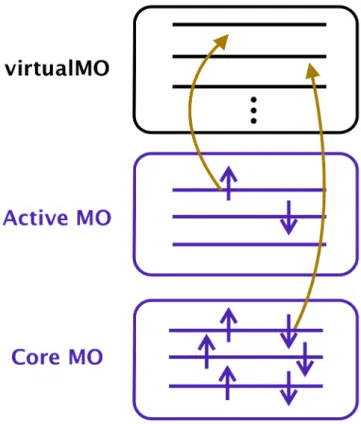Figure 1.1: The MO classification in the CAS framework. The core and virtual MOs are doubly occupied and unoccupied, respectively while the occupation number of the active MOs can be a decimal fraction ranging from 0 to 2.

## Introduction

This dependence essentially arises from the underlying full configurational expansion of the active-space wavefunction. The IC approach allows for rather simplified treatments of the intrinsic high-dimensional entanglement of the active-space wavefunction.

## Theory

• Notation and conventions
• The MRCI theory
• The IC-MRCI framework
• Commutator-based reduction scheme of higher rank RDMs
• Approximation to 4-RDM by cumulant reconstruction
• Diagonalization
• The IC-ACPF and IC-AQCC

Let us consider the diagonal blocks of the Hamiltonian matrix elements for the semi-interior basis IC Eikma (ooov) and the interior Ewikm (cooo), which are given by. The action of the Hamiltonian H on the reference function is written as. where Ψres refers to the residual interaction component.

## Implementation

### Automated tensor generation and implementation

Because of this factorization, the number of tensor contraction terms that actually involve the 4-RDM in the σ-vector. The explicit formulas of FIC-cu(4)-MRCI are attached to this article as supplementary material.

Truncation of the IC basis: Avoidance of variational collapse

## Benchmark sets and application

• Polyene: C n H n+2 , CAS(ne,no)
• The basis set dependence
• Free-base porphyrin: Singlet-triplet gap
• Comparison to WK-MRCI
• Comparison to full CI energy

In contrast, the computation time of FIC-MRCI increased rather slowly in polynomial order. To evaluate the accuracy of DMRG-cu(4)-MRCI, we calculated the energy gap between the lowest singlet and triplet states of the free base porphyrin molecule (C20H14N4).

## Conclusion

The computation time for FIC-MRCI is of polynomial order in the molecular size, whereas the WK and CW variants scale exponentially with increasing complexity of the variational space. Yet the cumulant approximation to 4-RDM often causes a variational breakdown because the neglect of the cumulants violates N-representability. For practical applications, the use of the DF technique is under investigation to improve the efficiency of our code.

The times for the intermediate construction ΓB [Eq. 2.57)], which need to be evaluated only once in the FIC-cu(4)-MRCI calculation, are also presented. CAS(6e, 6o) consisting of all 2p orbitals of N atoms was used for the reference space while the 1s orbitals were frozen. CAS(8e, 8o) consisting of all 2s and 2p orbitals of N atoms was used for reference while the 1s orbitals were frozen.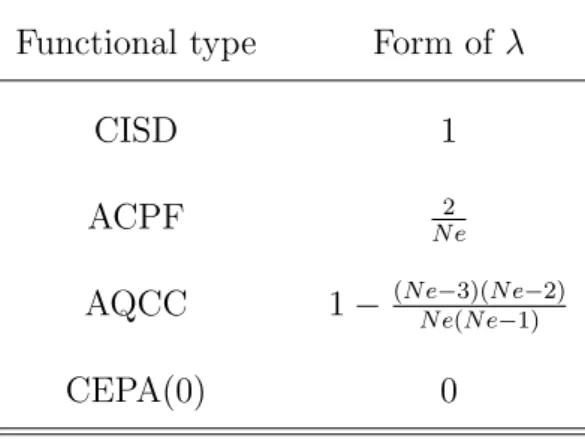Table 2.3: Forms of the factor λ, used in the correlation energy functionals.

## Introduction

In order to propose a theoretical question of the existence of the hypothetical iron(V)-oxoelectromere Cpd I, Pierloot et al. It was further suggested that in a second-order perturbative treatment, the dynamical correlation for the high-spin pentaradicaloid form of the iron(IV)-oxo electrometer would be overestimated. Therefore, the applicability of the conventional CAS method is limited to the outermost active space with 16 electrons distributed in 16 molecular orbitals (MOs).

By considering DMRG, the exact diagonalization of the active space Hamiltonian with about 30 – 40 active MOs is no longer an obstacle for multireference quantum chemistry. The use of the DMRG active space is key to performing such an extremely large-scale CAS treatment, which is far beyond the reach of conventional CAS methodologies. So far, the inclusion of dynamic correlation on top of the DMRG reference function has been possible through three approaches; (1) second-order multireference perturbation [DMRG-CASPT, multireference CT theory [DMRG-CT] and (3) MRCI and its variants corrected for size consistency, such as the coupled pair mean function (mean squared ACPF coupled cluster ( AQCC, which we call DMRG-MRCI, DMRG-ACPF and DMRG-AQCC respectively ).

## Theory

### The DMRG-MRCI

Because of this, the formal scaling of the DMRG-MRCI calculation is reduced to O(N8) from the previous one (O(N9)), where N refers to the size of the molecular size. 3.1), the action of the E operator on the reference IC generates the basis, an alternative many-body basis for the Slater determinant. The non-trivial conversion from Eq. 3.5) is responsible for eliminating the presence of 5-RDM without any approximation.

However, eight-index 4-RDM still hinders the application of DMRG-MRCI to systems with approximately 20 – 30 active MOs or more. The definition of the cumulant decomposition for the spin-free RDM is not as obvious as that in the spin-dependent case. The created program can use more than hundreds of computer nodes based on the parallelism of the message passing interface (MPI).

### Symbolic optimization of the cumulant-approximated 4- RDM

In the previous implementation of DMRG-MRCI, the 4-RDM is constructed on the fly from the lower-order RDM to satisfy the postulate. In this scheme, the cumulant 4-RDM reconstruction and the associated tensor contraction require floating-point operations (FPO) for O(N8) and O(N9), respectively. An optimization algorithm for tensor pre-sorting is implemented in the code generator.

Next, in this section we briefly outline the basic design of our tensor contraction program used for the construction of the σ vector. In this case, factoring the expression into the binary contraction and merging the loops shared by both binary contractions is quite simple. However, if the number of tensor factors is four, the number of unique combinations to form the binary contractions is as many as fifteen.

## Results and discussion

In this study, the electronic structure of the dimeric ferrate molecule is characterized using the DMRG method. We investigated the dependence of accuracy of the multireference treatments on the size of active space. Note that the use of the DFT(B3LYP) geometries for the DMRG calculations needs to be validated in future work.

We then continue with the qualitative characterization of the role of electrons in O-O bond formation. 4.5, the singly occupied 3dxz (3dyz) of each Fe is coupled to the doubly occupied 2px (2py) orbital of the corresponding O-ligand. 4.7, a general mechanism of the electronic process in the diferrate-mediated O-O bond reaction is schematically depicted.

Another branch of coupled-cluster (CC) methodologies  for the excited state, the single- and double-amplitude coupled equation-of-motion cluster (EOM-CCSD) [11, 12] and the fully renormalized triple-corrected version (CREOM-CCSD(T)) [ 13, 14] were also applied to ZnP and showed good agreement with the observed spectra . Furthermore, the second-order density matrix ρ(2) is given as. according to occupied/occupied space.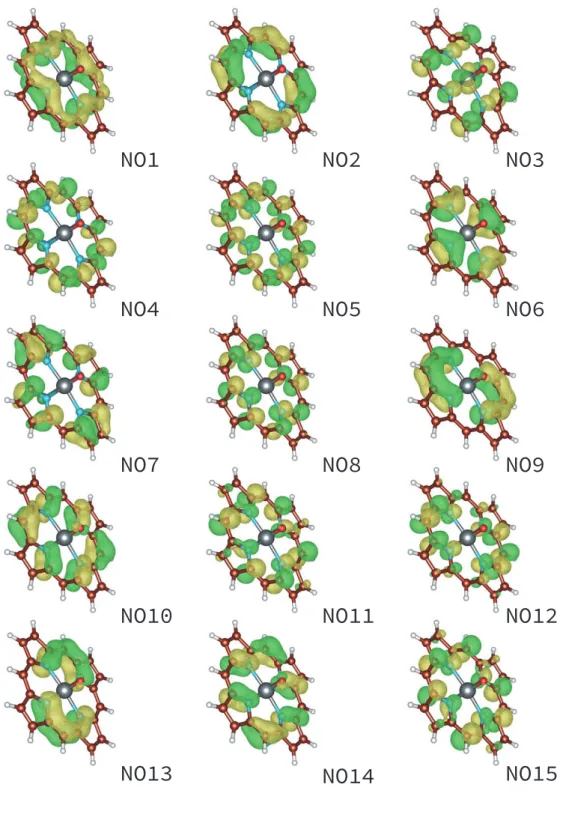Figure 3.1: The one-dimensional DMRG ordering of the natural orbitals (NOs), which were used to form the lattice sites of the DMRG state

Conclusing remarks

## Theoretical methods

### Ab initio density matrix renormalization group

The level of approximation is thus defined by M and is usually chosen to be in the range of several hundreds to thousands for molecular electronic structure calculations. The DMRG method is inherently particularly efficient for describing 1D many-body systems, as eqn (4.1) succinctly underlines the 1D nature of the DMRG representation. Challenging applications for such complex chemical systems can be found in previous DMRG studies on the tetranuclear manganese cluster of dicopper-dioxygen isomers, and 2Fe-2S ferredoxins. This capability significantly overcomes the limitation of conventional approaches.

In general, additional complexity for calculation setups arises in the ab initioDMRG approach; users are prompted to specify the shapes of the orbitals and their ordering order on the 1D grid. The use of these atomic-orbital (AO)-like MOs is likely to improve the feasibility of orbital ordering. The variational nature of the DMRG energy is useful for determining the shapes and orders of the orbitals.

Active space DMRG model

### DMRG-CASPT2 and DMRG-MRCI methods

Their description can be efficiently achieved with a lower-level theory such as configuration interaction perturbation theory,[79, 80] exponential-based transformation,[97, 101] etc. In this study, the complete second-order perturbation of the active space (CASPT2) )[ 92, 93] and multireference configuration interaction (MRCI). CASPT2 was introduced by Roos et al.[79, 80] as the multireference extension of the second-order Møller-Plesset theory.

Given the DMRG-CASSCF description as the zero-order wave function|Ψ(0)i, the DMRG-CASPT2 method determines the first-order perturbative correction for it, denoted |Ψ(1)i, as given by,. The most computationally intensive part of the DMRG-CAPST2 calculations lies in the estimations of the 3- and 4-particle reduced order density matrices (RDM) from the DMRG active space wave function. Our DMRG-MRCI implementation includes a posterior consistency magnitude correction, also known as the Davidson (+Q) correction.

## Computational details

Density functional theory (DFT) calculations

### DMRG-CASSCF/CASPT2/MRCI calculations

The localized orbitals obtained by the DMRG-CASSCF calculation for the reactant structure are shown in Figure S1†in order of our DMRG orbital ordering. Different sets of orbital orders were used for three segments of the reaction path as a function of the O-O distance: R(O-O). The dynamic correlation was evaluated with DMRG-CASPT2 and DMRG-MRCI+Q on top of the previous DMRG-CASSCF description.

In the DMRG-CASPT2 calculations, the modification to the zeroth-order Hamiltonian was used with the ionization potential/electron affinity (IPEA) specified as 0.25 a.u. The DMRG-MRCI+Q calculations used the overlap truncation with the threshold of τ = 5×10−2 (see also ref  for the definition of τ). The three-particle rank RDM was evaluated directly using the DMRG wave function, while the four-particle rank RDM was evaluated in an approximate manner using lower particle rank RDMs through the cumulant reconstruction neglecting the coupled four-rank cumulants.[94 , 116].

Conventional CASPT2/MRCI calculations

## Results and Discussions

Therefore, unless otherwise noted, our description of the natural orbitals in the O-O bonding process is given below at the CASSCF(20e,14o) level of theory. In our calculation for R, the oxidation states of Fe and O ions are confirmed to be +6 and -2, respectively. As the reaction proceeds from R to TS, the strength of the O-O interaction increases to the extent that the positions of σd−p.

The presence of an approximately doubly occupied σd−p orbital indicates that the σ-type bond is formed to a significant extent. It was found that the rate of σ bond formation is much faster than the rate of π bond formation. As shown in our scheme (Figure 4.7), the metal-oxo bonds are cleaved homolytically and the oxidation states of the Fe ions are reduced.

## Conclusions

The computational effort of DMRG-MRCI has been shown to scale polynomially with respect to the size of the system and the dimension of the active space. In Chapter 2, the derivation and implementation of DMRG-MRCI in an efficient vectorized program is given. Additionally, excited state calculations based on a hybrid of density-functional theory and multi-.

Moreover, the solution of the secular equation, Eq. A.9), X and Ω inherently satisfy size consistency . Therefore, the correlation level and polynomial scaling of the ADC(2) are the same. fully consistent with the MP2 ground state theory. Table A.2 shows the calculated excitation energies for the three types of porphyrin derivatives.

The symmetry of the associated excited states and the spectral signatures are also shown in Table A.2. ADC(2) can be considered as one of the quasi-degenerate perturbative variants of CIS(D) and the corresponding singly excited eigenvectors for such bands are obviously rotated due to the effect of strong quasi-degeneracy.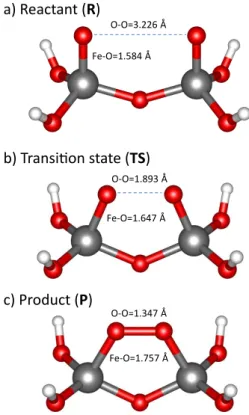Figure 4.1: Optimized structures for reactant (R), transition state (TS), and product (P) of diferrate, [H 4 Fe 2 O 7 ] 2+ .

## Theory

A summary of the ADC(2) model

Computational consideration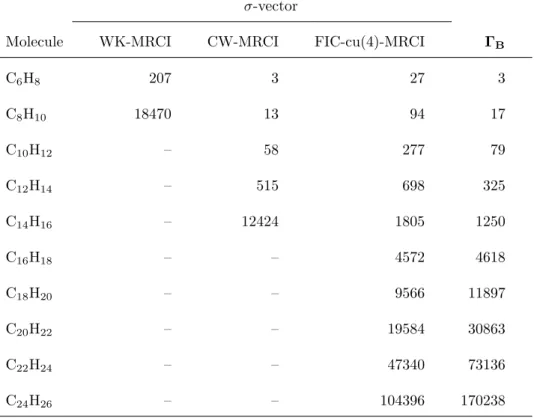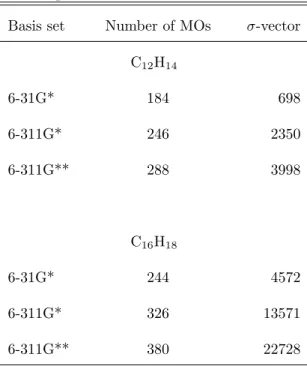+7

コエンザイム Q の微生物生産の実例と課題 島根大学 生物資源科学部 川向 誠 コエンザイムＱ（CoQ）はユビキノンとも称される脂溶性成分であり、生体内におい て電子伝達系の必須成分として重要な機能を果たしている。コエンザイムＱの側鎖部 分が10個のイソプレン単位からなるものをCoQ10と言い、最近サプリメントとして絶

192, p 111–116,（2010） 2） Motomura K, Ikeda T, Matsuyama S, Abdelhamid MAA, Tanaka T, Ishida T, Hirota R, Kuroda A The C-terminal zwitterionic sequence of CotB1 is essential for

Consistent with this, and building on the expanded Memorandum on Defence Cooperation see Attachment 1 signed between the Japanese Ministry of Defense and the Australian Ministry of

A China Watcher’s Perspective China unable and unwilling to accept the Senkaku Islands are not part of Taiwan Contradictions in China’s stance revealed in historical documents and

The purpose of the UI Team, which produced five papers, is to share the experiences of the economies where UI has already been introduced and to consider the implications for the

[r]

Here, we hypothesize that natural selection works to keep newly divergent gene pairs physically away, because their coregulation may be deleterious and/or because it takes a long

The main four contents in this article are: a constructing Ellis solutions in higher- dimensional general relativity, b stability analysis using linear perturbation method , c